### Hyperelastic Materials: Elasticity

In the energy section, we showed that the rate of change of the internal energy in a continuum under isothermic conditions is calculated using the “stress power” as follows: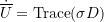If we assume that the energy during any reversible process is independent of the path, in other words, the energy supplied by the external loads on a deformable object is fully recoverable after the removal of the loads then the material is called elastic. “Hyperelastic Materials” or, alternatively, “Green Elastic Materials” are ideally elastic materials for which the stress-strain relationship derives from a strain energy potential function. For general elastic materials with large deformations accompanied by large volumetric changes, it is difficult to write a closed-form expression for the strain energy function in terms of the Cauchy stress since its energy conjugate is the stretching part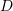of the velocity gradient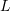, which is a rather instantaneous measure of deformation. Additionally, the small infinitesimal strain measure is not adequate when a continuum body exhibits large rotations. In fact, many homogeneous isotropic elastic materials exhibiting large deformations possess a nonlinear relationship between the stress and the strain measures used. In such materials, the strain energy density function is generally written in terms of the principal stretches or the singular values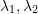, and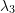of the deformation gradient.